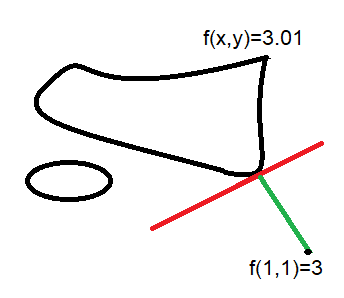# Good approximation - multivariable calculus

Poetria
Homework Statement:
##f(x,y) = x^3+x*y^2+y^3##
Then
f(1,1)=3
##f_x (1,1) = 4##
##f_y (1,1) = 5##
##\nabla f(1,1) = (4,5)##

Find the closest point to (1,1) at f(x,y) = 3.01, i.e. ##(1+\Delta x, 1+\Delta y)##
Relevant Equations:
Linear approximation
I tried to use a Taylor series expanded at 3 and set to 3.01:
https://www.wolframalpha.com/input/?i=27+++9+(-3+++x)^2+++(-3+++x)^3+++3+y^2+++y^3+++(-3+++x)+(27+++y^2)=3.01

I got the vector ## (\Delta x, \Delta y)= (0.37887, -0.54038)##
It does give a desired result but it is marked as wrong.

There is also a question about what statement is correct:

##(1+\Delta x, 1 + \Delta y)## is nearly parallel or perpendicular to ##(4,5)##
Or
##(\Delta x, \Delta y)## is nearly parallel or perpendicular to ##(4,5)##

I thought the vector (0.37887, -0.54038) was nearly perpendicular. Well...

Mentor
2022 Award
Homework Statement:: ##f(x,y) = x^3+x*y^2+y^3##
Then
f(1,1)=3
##f_x (1,1) = 4##
##f_y (1,1) = 5##
##\nabla f(1,1) = (4,5)##

Find the closest point to (1,1) at f(x,y) = 3.01, i.e. ##(1+\Delta x, 1+\Delta y)##
Relevant Equations:: Linear approximation

I tried to use a Taylor series expanded at 3 and set to 3.01:
https://www.wolframalpha.com/input/?i=27+++9+(-3+++x)^2+++(-3+++x)^3+++3+y^2+++y^3+++(-3+++x)+(27+++y^2)=3.01

I got the vector ## (\Delta x, \Delta y)= (0.37887, -0.54038)##
It does give a desired result but it is marked as wrong.

There is also a question about what statement is correct:

##(1+\Delta x, 1 + \Delta y)## is nearly parallel or perpendicular to ##(4,5)##
Or
##(\Delta x, \Delta y)## is nearly parallel or perpendicular to ##(4,5)##

I thought the vector (0.37887, -0.54038) was nearly perpendicular. Well...
Imaging we look at the surface ##(x,y,f(x,y))## from the top. Then it looks likeHence we are looking for a tangent spaces to the curve ##\{(x,y)\,|\,f(x,y)=3.01\}##. And then the one at ##(x_p,y_p)## among them for which tangent space and ##(1-x_p,1-y_p,3.01)## are perpendicular.

•Poetria
Poetria
I am not sure if I have understood you.
In that case, wouldn't the vector (1.44385, -1.44385) be a better solution?

I have tried to use

Mentor
2022 Award
I cannot guess the numbers and haven't made the calculation.

If you want to approach it numerically, then minimize the distance between ##(1,1,3)## and ##(x,y,3.01)## under the condition that ##x^3+xy^2+y^3=3.01##.

•Poetria
Poetria
I cannot guess the numbers and haven't made the calculation.

If you want to approach it numerically, then minimize the distance between ##(1,1,3)## and ##(x,y,3.01)## under the condition that ##x^3+xy^2+y^3=3.01##.
Thank you very much. I will try.

Poetria
This is the formula for the distance squared but I have no idea how to add the constraint:
##(1-x)^2+(1-y)^2+(3.01-3)^2##

Mentor
2022 Award
This is the formula for the distance squared but I have no idea how to add the constraint:
##(1-x)^2+(1-y)^2+(3.01-3)^2##
The keyword for approximations under constraints is the Lagrange multiplier. It is a technique to solve such problems. Wikipedia explains it quite well.

If you want to follow your path, then you'll get an equation ##g(x,y)=x^2+y^2+ax+by+c## which represents a paraboloid (opened at top). The solution will be its minimum.

Last edited:
•Frabjous, sysprog and Poetria
Homework Helper
Gold Member
2022 Award
I don't think anything too complicated is required. See if these (non-mathematician's) thoughts helps.

Point 1

Think of f(x,y) as a formula for the height of a hill, the xy plane being sea-level (ignore earth’s curvature). And imagine you have a map with contour lines.

Do know that ∇f(x,y) is a vector which points in the direction of ‘steepest ascent’ (maximum rate of change of f)?

If you are at P, above (1,1), your height is 3. Now imagine a contour line on your map for height = 3.01. You want the shortest way to get from P to the 3.01 contour line.

The shortest route will be the steepest – so your height will change most rapidly. The direction of the steepest route is given by vector ∇f(1,1) which is <4,5>. You need to move such that when your x-position changes 4 units, your y-position changes by 5 units.

That means Δy = (5/4)Δx

(We’re assuming ∇f(x,y) doesn’t change significantly while you move; it remains <4,5>. This is valid for small enough movements).
__________

Point 2

Have you met the ‘total differential’ (or ‘total derivative’)? In this case it is given by:

##df = \frac {∂f}{∂x}dx + \frac {∂f}{∂y}dy##

It gives the change in f resulting from changes in x and y. We are using Δx and Δy, so providing these values are small enough we can write:
##Δf = \frac {∂f}{∂x}Δx + \frac {∂f}{∂y}Δy##

Note that we we have Δf = 3.01 – 3.00 = 0.01

Edit: typo' corrected.

Last edited:
••Poetria and Delta2
Poetria
•Steve4Physics